Courses

# Notes | EduRev

## Class 10 : Notes | EduRev

The document Notes | EduRev is a part of the Class 10 Course Class 10 Mathematics by VP Classes.
All you need of Class 10 at this link: Class 10

Q.1. In the figure, PS is the bisector of ∠QPR of Δ PQR. Prove that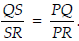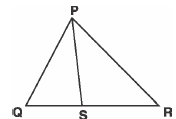Sol.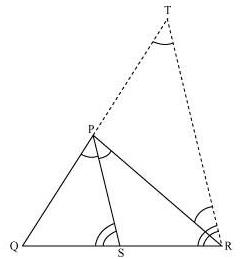Let us draw a line segment RT parallel to SP which intersects extended line segment QP at point T.
Given that, PS is the angle bisector of ∠QPR.
∠QPS = ∠SPR … (1)
By construction,
∠SPR = ∠PRT (As PS || TR) … (2)
∠QPS = ∠QTR (As PS || TR) … (3)
Using these equations, we obtain
∠PRT = ∠QTR
∴ PT = PR
By construction,
PS || TR
By using basic proportionality theorem for ΔQTR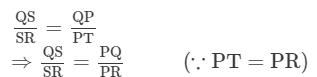Q.2. In the figure, D is a point on hypotenuse AC of ΔABC, such that BD ⊥ AC, DM BC and DN AB.
Prove that:
(i) DM2 = DN.MC
(ii) DN2 = DM.AN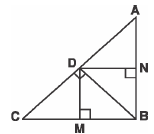Sol.
(i) Let us join DB.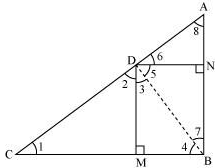We have, DN || CB, DM || AB, and ∠B = 90°
∴ DMBN is a rectangle.
∴ DN = MB and DM = NB
The condition to be proved is the case when D is the foot of the perpendicular drawn from B to AC.
∴ ∠CDB = 90°
⇒ ∠2 + ∠3 = 90° … (1)
In ΔCDM,
∠1 + ∠2 + ∠DMC = 180°
⇒ ∠1 + ∠2 = 90° … (2)
In ΔDMB,
∠3 + ∠DMB + ∠4 = 180°
⇒ ∠3 + ∠4 = 90° … (3)
From equation (1) and (2), we obtain
∠1 = ∠3
From equation (1) and (3), we obtain
∠2 = ∠4
In ΔDCM and ΔBDM,
∠1 = ∠3 (Proved above)
∠2 = ∠4 (Proved above)
∴ ΔDCM ∼ ΔBDM (AA similarity criterion)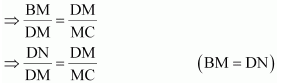⇒ DM2 = DN × MC
(ii) In right triangle DBN,
∠5 + ∠7 = 90° … (4)
In right triangle DAN,
∠6 + ∠8 = 90° … (5)
D is the foot of the perpendicular drawn from B to AC.
⇒ ∠5 + ∠6 = 90° … (6)
From equation (4) and (6), we obtain
∠6 = ∠7
From equation (5) and (6), we obtain
∠8 = ∠5
In ΔDNA and ΔBND,
∠6 = ∠7 (Proved above)
∠8 = ∠5 (Proved above)
∴ ΔDNA ∼ ΔBND (AA similarity criterion)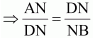⇒ DN2 = AN × NB
⇒ DN2 = AN × DM (As NB = DM)

Q.3. In the figure, ABC is a triangle in which ∠ABC >90° and AD ⊥ CB produced. Prove that AC2 = AB2 + BC2 + 2 BC.BD.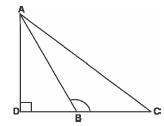Sol. Applying Pythagoras theorem in ΔADB, we obtain
AB2 = AD2 + DB2 … (1)
Applying Pythagoras theorem in ΔACD, we obtain
AC2 = AD2 + (DB + BC)2
AC2 = AD2 + DB2 + BC2 + 2DB × BC
AC2 = AB2 + BC2 + 2DB × BC [Using equation (1)]
Thus, we have:
AC2 = AB+ BC2 + 2 BC·BD

Q.4. In the figure, ABC is a triangle in which ABC 90° and AD ⊥ BC. Prove that AC2 = AB2 + BC2 - 2 BC.BD.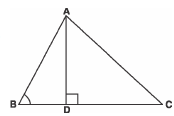Sol. Applying Pythagoras theorem in ΔADB, we obtain
⇒ AD2 = AB2 − DB2 … (1)
Applying Pythagoras theorem in ΔADC, we obtain
AB2 − BD2 + DC2 = AC2 [Using equation (1)]
AB2 − BD2 + (BC − BD)2 = AC2
AC2 = AB2 − BD2 + BC2 + BD2 − 2BC × BD
= AB2 + BC2 − 2BC × BD

Q.5. In the figure, AD is a median of a triangle ABC and AM BC. Prove that: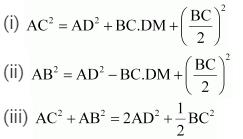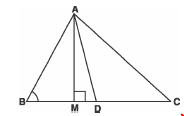Sol. (i) Applying Pythagoras theorem in ΔAMD, we obtain
AM2 + MD2 = AD2 … (1)
Applying Pythagoras theorem in ΔAMC, we obtain
AM2 + MC2 = AC2
AM2 + (MD + DC)2 = AC2
(AM2 + MD2) + DC2 + 2MD.DC = AC2
AD2 + DC2 + 2MD.DC = AC2 [Using equation (1)]
Using the result, DC = BC/2 , we obtain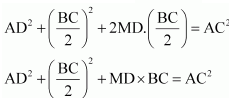(ii) Applying Pythagoras theorem in ΔABM, we obtain
AB2 = AM2 + MB2
= (AD2 − DM2) + MB2
= (AD2 − DM2) + (BD − MD)2
= AD2 − DM2 + BD2 + MD2 − 2BD × MD
= AD2 + BD2 − 2BD × MD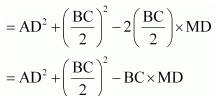(iii) Applying Pythagoras theorem in ΔABM, we obtain
AM2 + MB2 = AB2 … (1)
Applying Pythagoras theorem in ΔAMC, we obtain
AM2 + MC2 = AC2 … (2)
Adding equations (1) and (2), we obtain
2AM2 + MB2 + MC2 = AB2 + AC2
2AM2 + (BD − DM)2 + (MD + DC)2 = AB2 + AC2
2AM2 +BD2 + DM2 − 2BD.DM + MD2 + DC2 + 2MD.DC = AB2 + AC2
2AM2 + 2MD2 + BD2 + DC2 + 2MD (− BD + DC) = AB2 + AC2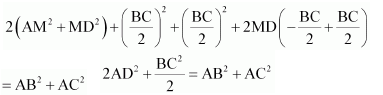Q.6. Prove that the sum of the squares of the diagonals of parallelogram is equal to the sum of the squares of its sides.
Sol.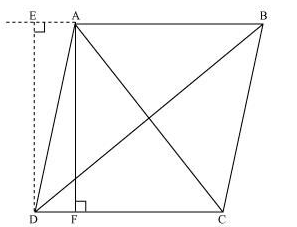Let ABCD be a parallelogram.
Let us draw perpendicular DE on extended side AB, and AF on side DC.
Applying Pythagoras theorem in ΔDEA, we obtain
DE2 + EA2 = DA2 … (i)
Applying Pythagoras theorem in ΔDEB, we obtain
DE2 + EB2 = DB2
DE2 + (EA + AB)2 = DB2
(DE2 + EA2) + AB2 + 2EA × AB = DB2
DA2 + AB2 + 2EA × AB = DB2 … (ii)
Applying Pythagoras theorem in ΔADF, we obtain
Applying Pythagoras theorem in ΔAFC, we obtain
AC2 = AF2 + FC2
= AF2 + (DC − FD)2
= AF2 + DC2 + FD2 − 2DC × FD
= (AF2 + FD2) + DC2 − 2DC × FD
AC2 = AD2 + DC2 − 2DC × FD … (iii)
Since ABCD is a parallelogram,
AB = CD … (iv)
And, BC = AD … (v)
∠DEA = ∠AFD (Both 90°)
∴ ΔEAD  ΔFDA (AAS congruence criterion)
⇒ EA = DF … (vi)
Adding equations (i) and (iii), we obtain
DA2 + AB2 + 2EA × AB + AD2 + DC2 − 2DC × FD = DB2 + AC2
DA2 + AB. + AD2 + DC2 + 2EA × AB − 2DC × FD = DB2 + AC2
BC2 + AB2 + AD2 + DC2 + 2EA × AB − 2AB × EA = DB2 + AC2
[Using equations (iv) and (vi)]
AB2 + BC2 + CD2 + DA2 = AC2 + BD2

Q7. In the figure, two chords AB and CD intersect each other at the point P. Prove that:
(i) Δ APC ~ Δ DPB

(ii) AP·PB = CP·DP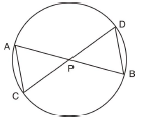Sol. Let us join CB.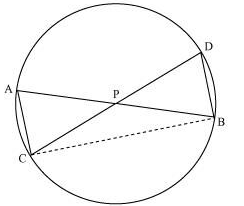(i) In ΔAPC and ΔDPB,
∠APC = ∠DPB (Vertically opposite angles)
∠CAP = ∠BDP (Angles in the same segment for chord CB)
ΔAPC ∼ ΔDPB (By AA similarity criterion)
(ii) We have already proved that
ΔAPC ∼ ΔDPB
We know that the corresponding sides of similar triangles are proportional.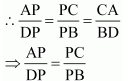Q.8. In the figure, two chords AB and CD of a circle intersect each other at the point P (when produced) outside the circle.
Prove that
(i) Δ PAC ~ Δ PDB
(ii) PA·PB = PC·PD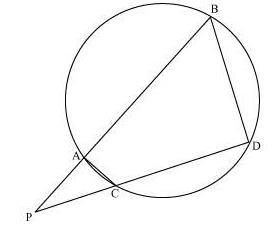Sol.
(i) In ΔPAC and ΔPDB,
∠P = ∠P (Common)
∠PAC = ∠PDB (Exterior angle of a cyclic quadrilateral is ∠PCA = ∠PBD equal to the opposite interior angle)
∴ ΔPAC ∼ ΔPDB
(ii)We know that the corresponding sides of similar triangles are proportional.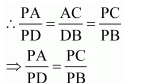∴ PA.PB = PC.PD

Q.9. In the figure, D is a point on side BC of Δ ABC such that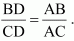Prove that AD is the bisector of ∠BAC.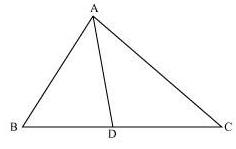Sol. Let us extend BA to P such that AP = AC. Join PC.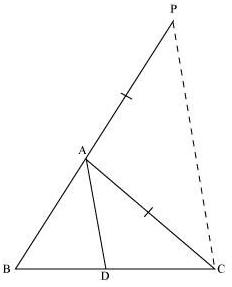It is given that,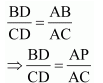By using the converse of basic proportionality theorem, we obtain
⇒ ∠BAD = ∠APC (Corresponding angles) … (1)
And, ∠DAC = ∠ACP (Alternate interior angles) … (2)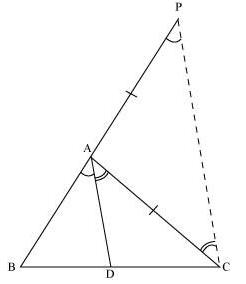By construction, we have
AP = AC
⇒ ∠APC = ∠ACP … (3)
On comparing equations (1), (2), and (3), we obtain
⇒ AD is the bisector of the angle BAC.

Q.10. Nazima is fly fishing in a stream. The tip of her fishing rod is 1.8 m above the surface of the water and the fly at the end of the string rests on the water 3.6 m away and 2.4 m from a point directly under the tip of the rod. Assuming that her string (from the tip of her rod to the fly) is taut, how much string does she have out (see figure)? If she pulls in the string at the rate of 5 cm per second, what will be the horizontal distance of the fly from her after 12 seconds?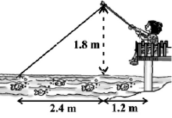Sol.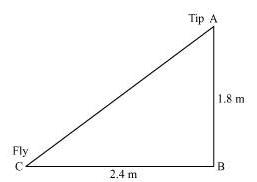Let AB be the height of the tip of the fishing rod from the water surface. Let BC be the horizontal distance of the fly from the tip of the fishing rod.
Then, AC is the length of the string.
AC can be found by applying Pythagoras theorem in ΔABC.
AC2 = AB2 + BC2
AB2 = (1.8 m)2 + (2.4 m)2
AB2 = (3.24 + 5.76) m2
AB2 = 9.00 m2
Thus, the length of the string out is 3 m.
She pulls the string at the rate of 5 cm per second.
Therefore, string pulled in 12 seconds = 12 × 5 = 60 cm = 0.6 m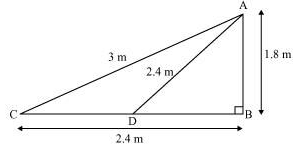Let the fly be at point D after 12 seconds.
Length of string out after 12 seconds is AD.
AD = AC − String pulled by Nazima in 12 seconds
= (3.00 − 0.6) m
= 2.4 m
(1.8 m)2 + BD2 = (2.4 m)2
BD2 = (5.76 − 3.24) m2 = 2.52 m2
BD = 1.587 m
Horizontal distance of fly = BD + 1.2 m
= (1.587 + 1.2) m
= 2.787 m
= 2.79 m

Offer running on EduRev: Apply code STAYHOME200 to get INR 200 off on our premium plan EduRev Infinity!

132 docs

,

,

,

,

,

,

,

,

,

,

,

,

,

,

,

,

,

,

,

,

,

;